# C++ Tutorial With Basic Programs for Beginners

C++ tutorial, C++ programs, C++ code, C++ flowcharts, C++ statements, C++ syntax, C++ examples

## Monday, May 27, 2019

### Write a program in C language to print all prime numbers between 1 and a given number n input by user at run time.

This C program will display all prime numbers from 1 to a user supplied  number n.
// cprime, prime in c, prime series in c, n prime

// C++ program to input a number n
//then prints prime number series from 1 to n
//that is prints all prime between 1 to n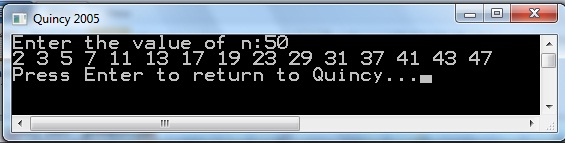cprime-numbers-series-from-1-to-N-program-source-code

#include <iostream>
using namespace std;

int checkPrimeNumber(int);

int main() {
bool isPrime;
int n, num;
cout<<"Enter the value of n:";
cin>>num;
for(int n = 2; n < num; n++)
{
// if n is prime then boolean variable isPrime will be true
isPrime = checkPrimeNumber(n);

if(isPrime == true)
cout<<n<<" ";
}
return 0;
}

// Function that checks whether n is prime or not
int checkPrimeNumber(int n) {
bool isPrime = true;

for(int i = 2; i <= n/2; i++) {
if (n%i == 0)
{
isPrime = false;
break;
}
}
return isPrime;
}

//Output:

//Enter the value of n:50
//2 3 5 7 11 13 17 19 23 29 31 37 41 43 47

Share:

## Saturday, March 24, 2018

### Easyway How To Write a Program in C++ for Calculating Area of Triangle When base and height is given

Program Statement: Write a C++ program to input base and height of a triangle and calculates and prints the area of triangle.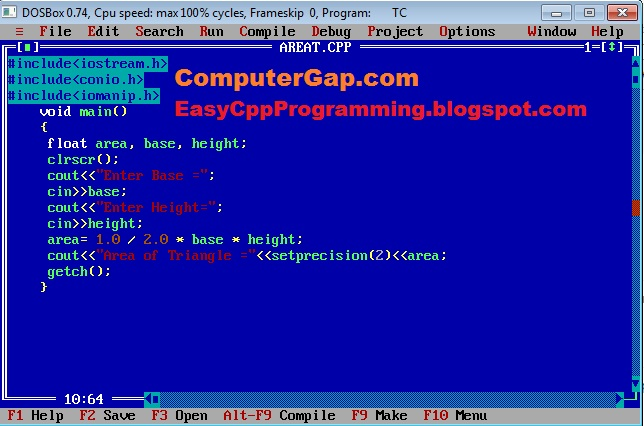Input base and height, compute area of triangle c++ program

### Formula for Area of Triangle C++ program when base and height are given

The formula for area of triangle to be used in this C++ program is as as follows:
1. area of triangle = 1 / 2 x base x height

#### How Formula of  Area of Triangle is Transformed into C++ Arithmetic Expressions

In C++ programming, we write the above formula as following C++ expression.

#### area = 1.0/2.0 * base * height

or we can also use the formula: area = 0.5 * base * height

### Source Code for Modern C++ Compilers / IDEs

```#include<iostream>
#include<iomanip>
using namespace std;
int main()
{

float area, base, height;

cout<<"Enter base  = ";
cin>>base;
cout<<"Enter height  = ";
cin>>height;

area = 1.0 / 2.0  * base * height;

cout<<"Area of  Triangle = "<<setprecision(2)<<area<<endl;
return 0;
}
```

### C++ Source Code for Calculating Area of Triangle When Base and Height are Given (for Turbo C++ 3.0 compiler / IDE)

#include<iostream.h>
#include<conio.h>
#include<iomanip.h>

void main()
{

float area, base, height;
clrscr();
cout<<"Enter base  = ";
cin>>base;
cout<<"Enter height  = ";
cin>>height;

area = 1.0 / 2.0  * base * height;

cout<<"Area of  Triangle = "<<setprecision(2)<<area<<endl;

getch();
}

### How This Program works : C++ Source Code for Calculating Area of Triangle When Base and Height are Given

Here is a detailed C++ program with simplified documentation included as C++ comments, so that the logic of C++ Program Area of Triangle can be understood by readers and other C++ programmers easily:

#include<iostream.h>   //include for cin, cout
#include<conio.h>     //include for clrscr()
#include<iomanip.h>  //include for setprecision()

void main()
{

float area, base, height;   //declare variables
clrscr();
cout<<"Enter base  = ";
cin>>base;                          //input base
cout<<"Enter height  = ";
cin>>height;      //input height

area = 1.0 / 2.0  * base * height;  //compute area

cout<<"Area of  Triangle = "<<setprecision(2)<<area<<endl; // show result

getch();
}

Output:
Enter Base: 5.2
Enter Height: 10.8
Area of Triangle = 28.08

Share:

### C++ Program Compute Sum of Cubes of Digits of User Supplied Number Easyway

#### Q: Write a Program in C++ Programming language that inputs an integer number and computes the sum of cubes of digits of  this user supplied number at run time.

For example, if given number is 123 then answer will be Sum of cubes of  digits = 36
because 13+23+33 = 1+8+27 = 36.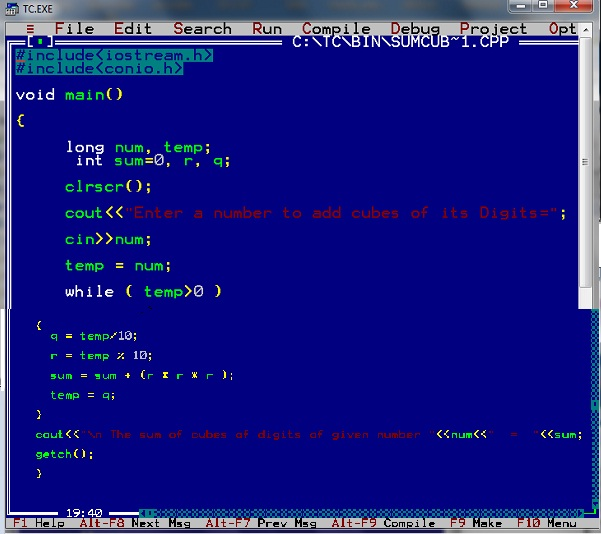C++ Program to compute sum of cubes of digits of user supplied number

### C++ Source Code of Sum of cubes of Digits of a given number Program

/*
Program to input a number, then display sum of cubes of its digits.
(c) Www.EasyCppProgramming.Blogspot.Com
*/
#include<iostream.h>
#include<conio.h>
#include<math.h>
void main()
{
long num, temp;
int, sum=0, r, q;
clrscr();
cout<<"Enter a number to add cubes of its Digits=";
cin>>num;
temp = num;
while ( temp>0 )
{
q = temp/10;
r = temp % 10;
sum = sum +  ( r * r * r);

temp = q;
}
cout<<"\n The sum of cubes of digits of given number "<<num<<"  =  "<<sum;
getch();
}Calculate-cubes-of-digits-of-user-given number

### The Working of  C++ Code of Add Digits of a given number Program

This program is of reverse number logic.

1. We will divide 123 by 10 and get quotient=12, r = 3
2. add r*r*r=3*3*3  into sum, so sum=27

3. Now put q =12 into dividend number
4. so divide 12 by 10, quotient=1 and remainder r=2.
5. Add r*r*r=2*2*2 into sum, so sum will become 27+8 =35.
6. now put q=1 into dividend number

7. Now divide 1 by 10, quotient=0 and r=1.
8. so add r*r*r=1*1*1 into sum, and sum becomes 35+1= 36
9. now put quotient=0 into dividend number called temp variable
10. so that while loop test condition while (temp>0) becomes false and loop terminates.

11. Therefore display the answer sum of cubes of digits of number 123 = 36

Share:

### C++ Program Calculate Sum of Squares of Digits of Given Number

#### Q: Write a C++ Program to input an integer number. Calculate the sum of squares of digits of          this number.

For example, if given number is 123 then answer will be Sum squares of  digits = 14
because 12+22+32 = 1+4+9 = 14.C++ Program to Calculate sum of squares of digits of given number

### C++ Code of Sum of Squares of Digits of a given number Program

/*
Program to input a number, then display sum of squares of its digits.
(c) Www.EasyCppProgramming.Blogspot.Com
*/
#include<iostream.h>
#include<conio.h>
#include<math.h>
void main()
{
int num, temp, sum=0, r, q;
clrscr();
cout<<"Enter a number to add square of its Digits=";
cin>>num;
temp = num;
while ( temp>0 )
{
q = temp/10;
r = temp % 10;
sum = sum +  ( r * r);

temp = q;
}
cout<<"\n The sum of squares of digits of given number "<<num<<"  =  "<<sum;
getch();
}

### The Working of  C++ Code of Add Digits of a given number Program

This program is of reverse number logic.

1. We will divide 123 by 10 and get quotient=12, r = 3
2. add r*r=3*3  into sum, so sum=9

3. Now put q =12 into dividend number
4. so divide 12 by 10, quotient=1 and remainder r=2.
5. Add r*r=2*2 into sum, so sum will become 9+4 =13.
6. now put q=1 into dividend number

7. Now divide 1 by 10, quotient=0 and r=1.
8. so add r*r=1*1 into sum, and sum becomes 13+1= 14
9. now put quotient=0 into dividend number called temp variable
10. so that while loop test condition while (temp>0) becomes false and loop terminates.

11. Therefore display the answer sum of squares of digits of number 123 = 14C++ Program to Calculate sum of squares of digits of given number execution

/*
Program to input a number, then display sum squares of its digits.
(c) Www.EasyCppProgramming.Blogspot.Com
*/
#include<iostream.h>     // include header files
#include<conio.h>

void main()
{
int num, temp, sum=0, r, q;
clrscr();
cout<<"Enter a number to add its Digits=";
cin>>num;                 // input number
temp = num;             // copy number into temp variable
while ( temp>0 )      // loop while temp is greater than zero
{
q = temp/10;      // calculate quotient = temp number / 10, if num is 123 then q=12
r = temp % 10;  //  calculate remainder r = 123 % 10, r = 3
sum = sum + ( r * r );  // sum = 0 + 3x3 =0+9
// so square of last digit 3 of 123 is added in sum  variable

temp = q;      // set next time dividend     temp=12
}
cout<<"\n The sum of digits of given number "<<num<<"  =  "<<sum; //sum=14
getch();
}
Share:

### C++ Program To Find Product of Digits of Given Number

#### Q: Input an integer number. Find and show the product / multiplication of digits of given number. That is we have to multiply digits of number.

For example, if given number is 123 then answer will be Product of digits = 6
because 1 x 2 x 3 = 6.C++ Program to calculate product of digits of a number

### The Actual C++ Code of C++ Program Product of Digits of a given number

/*
Program to input a number, then display poduct of its digits.
(c) Www.EasyCppProgramming.Blogspot.Com
*/

#include<iostream.h>
#include<conio.h>

void main()

{

int num, temp, product=1, r, q;

clrscr();

cout<<"Enter a number to multiply its Digits=";

cin>>num;

temp = num;

while ( temp>0 )

{
q = temp/10;

r = temp % 10;

product = product * r;

temp = q;

}

cout<<"\n The product of digits of given number "<<num<<" = "<<product;

getch();
}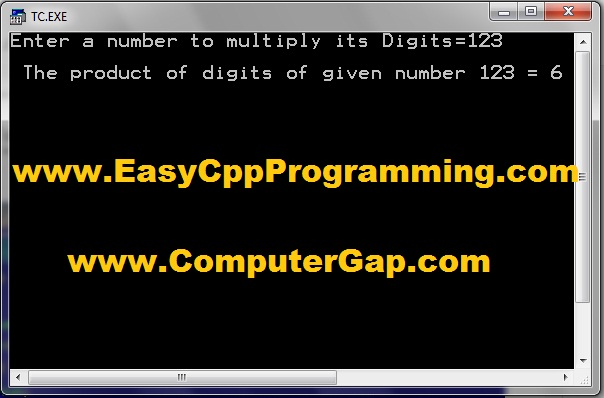C++ Program to calculate product of digits of a number

### The Working of  C++ Code of product of Digits of a given number Program

This program is of reverse number logic.

1. We will divide 123 by 10 and get quotient=12, r = 3
2. multiply r=3 with product which is one so product=3

3. Now put q =12 into dividend number
4. so divide 12 by 10, quotient=1 and remainder r=2.
5. Multiply r=2 with product which is 3 so product = 6.
6. now put q=1 into dividend number

7. Now divide 1 by 10, quotient=0 and r=1.
8. so multiply r=1 with product which is 6 therefore product becomes 6 x 1 = 6
9. now put quotient=0 into dividend number called temp variable
10. so that while loop test condition while (temp>0) becomes false and loop terminates.

11. Therefore display the answer product of digits of number 123 = 6
/*
Program to input a number, then display product of its digits.
(c) Www.EasyCppProgramming.Blogspot.Com
*/
#include<iostream.h>     // include header files
#include<conio.h>

void main()
{
int num, temp, product=1, r, q;
clrscr();
cout<<"Enter a number to multiply its Digits=";
cin>>num;                 // input number
temp = num;             // copy number into temp variable
while ( temp>0 )      // loop while temp is greater than zero
{
q = temp/10;      // calculate quotient = temp number / 10, if num is 123 then q=12
r = temp % 10;  //  calculate remainder r = 123 % 10, r = 3
product = produt * r;  // product = 1* 3; so last digit 3 of 123 is multiplied,
//product=3

temp = q;      // set next time dividend     temp=12
}
cout<<"\n The product of digits of given number "<<num<<"  =  "<<product;
//product=6
getch();
}
Share:

### C++ Program To Count Number of Digits in a Given Number

#### Q: Input an integer number. Find and show the COUNT of digits of given number.

For example, if given number is 123 then answer will be Count of digits = 3
because 123  consists of  3 digits.C++ Program to count number of digits in a given number

### The Actual C++ Code of Add Digits of a given number Program

/*
Program to input a number, then display count of its digits.
(c) Www.EasyCppProgramming.Blogspot.Com for Easyway C++ Programs
www.ComputerGap.com for perfect computer study notes
*/
#include<iostream.h>
#include<conio.h>

void main()

{

int num, temp, count=0, r, q;

clrscr();

cout<<"Enter a number to Count its Digits=";

cin>>num;

temp = num;

while ( temp>0 )

{
q = temp/10;

count++;

temp = q;

}

cout<<"\n The Count of digits of given number "<<num<<"  =  "<<count;

getch();

}

### Each and Every Statement Explained

This program is of reverse number logic.

1. We will divide 123 by 10 and get quotient=12, r = 3
2. increment count by 1 so count =1

3. Now put q =12 into dividend number
4. so divide 12 by 10, quotient=1 and remainder r=2.
5. increment count by 1, so count =2
6. now put q=1 into dividend number

7. Now divide 1 by 10, quotient=0 and r=1.
8. increment to count by 1 so count=3
9. now put quotient=0 into dividend number called temp variable
10. so that while loop test condition while (temp>0) becomes false and loop terminates.

11. Therefore display the answer count of digits of number 123 = 3C++ Program to count number of digits in a given number sample run output

/*
Program to input a number, then display count of its digits. with comments /documentation
(c) Www.EasyCppProgramming.Blogspot.Com
*/
#include<iostream.h>     // include header files
#include<conio.h>

void main()
{
int num, temp, sum=0, r, q;
clrscr();
cout<<"Enter a number to count its Digits=";
cin>>num;                 // input number
temp = num;             // copy number into temp variable
while ( temp>0 )      // loop while temp is greater than zero
{
q = temp/10;      // calculate quotient = temp number / 10, if num is 123 then q=12

count++ ;  // starting from 0, increment to count = 1

temp = q;      // set next time dividend     temp=12
}
cout<<"\n The Count of digits of given number "<<num<<"  =  "<<count;     //count=3
getch();
}

Share:

### C++ Program To Find Sum of Digits of Given Number

#### Q: Input an integer number. Find and show the sum of digits of given number.

For example, if given number is 123 then answer will be Sum of digits = 6
because 1+2+3 = 6.Input Number Add its digits C++ Program

### The Actual C++ Code of Add Digits of a given number Program

/*
Program to input a number, then display sum of its digits.
(c) Www.EasyCppProgramming.Blogspot.Com
*/
#include<iostream.h>
#include<conio.h>
#include<math.h>
void main()
{
int num, temp, sum=0, r, q;
clrscr();
cout<<"Enter a number to add its Digits=";
cin>>num;
temp = num;
while ( temp>0 )
{
q = temp/10;
r = temp % 10;
sum = sum + r;

temp = q;
}
cout<<"\n The sum of digits of given number "<<num<<"  =  "<<sum;
getch();
}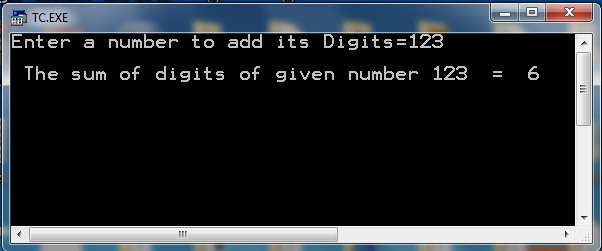Write C++ Program to find sum of digits of given number

### The Working of  C++ Code of Add Digits of a given number Program

This program is of reverse number logic.

1. We will divide 123 by 10 and get quotient=12, r = 3
2. add r=3 into sum, so sum=3

3. Now put q =12 into dividend number
4. so divide 12 by 10, quotient=1 and remainder r=2.
5. Add r=2 into sum, so sum will become 5.
6. now put q=1 into dividend number

7. Now divide 1 by 10, quotient=0 and r=1.
8. so add r=1 into sum, and sum becomes 6
9. now put quotient=0 into dividend number called temp variable
10. so that while loop test condition while (temp>0) becomes false and loop terminates.

11. Therefore display the answer sum of digits of number 123 = 6

/*
Program to input a number, then display sum of its digits.
(c) Www.EasyCppProgramming.Blogspot.Com
*/
#include<iostream.h>     // include header files
#include<conio.h>

void main()
{
int num, temp, sum=0, r, q;
clrscr();
cout<<"Enter a number to add its Digits=";
cin>>num;                 // input number
temp = num;             // copy number into temp variable
while ( temp>0 )      // loop while temp is greater than zero
{
q = temp/10;      // calculate quotient = temp number / 10, if num is 123 then q=12
r = temp % 10;  //  calculate remainder r = 123 % 10, r = 3
sum = sum + r;  // sum = 0 + 3; so last digit 3 of 123 is added in sum variable

temp = q;      // set next time dividend     temp=12
}
cout<<"\n The sum of digits of given number "<<num<<"  =  "<<sum; //sum=6
getch();
}
Share:

## Friday, March 23, 2018

### Easyway How To Write a Program in C++ for Calculating Area and Circumference of Circle

Program Statement: Write a C++ program to input radius of a circle and calculates and prints the area and circumference of the given circle.Calculate area and circumference of circle in C++ Program Easyway

### What are the Formulas Used in C++ Program for Area and Circumference of Circle?

To write this program, first of all we have to find the accurate formulas for area and circumference of circle.
These are as follows:
1. area of circle = Π R 2
2. circumference of circle =  2 Π R

#### How Formulas of Circle are Transformed into C++ Arithmetic Expressions

In C++ programming, we write the above formulas as follows
1. area = 3.14 * radius * radius
2. circum = 2 * 3.14 * radius
Note: 3.14 is the vale of constant PI  shown with symbol Π

A Better Programming Approach is to Use Symbolic Constants instead of hard coding numbers directly in C++ programs.

### for Calculating Area and Circumference of  Circle

There are two ways:
1. Using #define Pre processor Directive:     #define PI  3.14
2. Using const Qualifier :                             const float PI = 3.14;

### C++ Source Code for Calculating Area and Circumference of  Circle

#include<iostream.h>
#include<conio.h>
#include<iomanip.h>
#define PI 3.14
void main()
{

float radius, area, circum;
clrscr();
cout<<"Enter radius of circle = ";
cin>>radius;
area = PI * radius * radius;
circum = 2 * PI * radius;

cout<<"Area of  circle = "<<setprecision(2)<<area<<endl;
cout<<"Circumference of circle = "<<setprecision(2)<<circum;

getch();
}

### How This Program Works for Calculating Area and Circumference of  Circle

#include<iostream.h>  // include header file for cin, cout
#include<conio.h>     // include header file for  clrscr()
#include<iomanip.h>  // include header file for setprecision()
#define PI 3.14   // define constant PI
void main()
{

float radius, area, circum;   declare variables
clrscr();
cout<<"Enter radius of circle = ";
cin>>radius;           //input radius
area = PI * radius * radius;   // calculate area
circum = 2 * PI * radius;   // calculate circumference

// used setprecision(2) to show value of area upto two decimal places
cout<<"Area of  circle = "<<setprecision(2)<<area<<endl;

// used setprecision(2) to show value of circumference upto two decimal places
cout<<"Circumference of circle = "<<setprecision(2)<<circum;

getch();
}

Share:

### C++ Program to Calculate Bonus on Salary Grade Wise

Q: Write a C++ Program To Calculate Bonus on Salary Grade Wise

Program Statement: Write a C++ Program to input salary and grade. it applies a 60% bonus to employees of grade 17 or above. It adds a 30% bonus for all employees of grade less than 17.Salary Bonus C|++ Program

### Source code of Salary Program for Dev C++ and Modern C++ Compilers / IDEs

```#include<iostream>

using namespace std;

int main()
{
float salary,bonus;
int grade;

cout<<"Enter salary of Employee=";
cin>>salary;
cout<<"Enter grade=";
cin>>grade;

if(grade>=17)
bonus = salary * 60.0/100.0;
else
bonus = salary * 30.0/100.0;
salary = salary + bonus;
cout<<"The Total salary of Employee = "<<salary;

return 0;
}
```

## Sample output of Salary Grade Bonus Program

Enter salary of Employee=10000
Enter grade=17
The Total salary of Employee = 16000
--------------------------------
Process exited after 26.75 seconds with return value 0
Press any key to continue . . .

## C++ Source Code of  Program To Calculate Bonus on Salary for Turbo C++ 3.0 IDE / Compiler

#include<iostream.h>
#include<conio.h>

void main()
{
float salary,bonus;
int grade;
clrscr();
cout<<"Enter salary of Employee=";
cin>>salary;
cout<<"Enter grade=";
cin>>grade;

if(grade>=17)
bonus = salary * 60.0/100.0;
else
bonus = salary * 30.0/100.0;
salary = salary + bonus;
cout<<"The Total salary of Employee = "<<salary;

getch();
}

### Output of Sample Run: Salary Bonus C ++ Program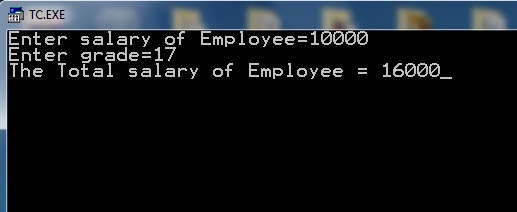Calculate Salary with Bonus according to Grade C++ Program

## How Salary Bonus C++ Program Works: Logic

#include<iostream.h>      //include header files
#include<conio.h>

void main()
{
float salary,bonus;
int grade;
clrscr();
cout<<"Enter salary of Employee=";
cin>>salary;                   // Enter salary
cout<<"Enter grade=";
cin>>grade;                   // input grade

// check if grade greater than or equal to 17

if(grade>=17)
bonus = salary * 60.0/100.0;    //  calculate 60% bonus
else
bonus = salary * 30.0/100.0; // calculate 30% bonus
salary = salary + bonus;     // add bonus to salary

// print salary
cout<<"The Total salary of Employee = "<<salary;

//for staying output window until the user presses a key  getch();
}

Share:

## Thursday, March 22, 2018

### Program Area of Triangle Algorithm Flowchart

Q: Write a C++ Program to input three sides of a triangle. The program then calculates the Area of triangle by the formula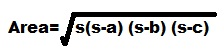Formula of area of triangle when 3 sides given
Where s = ( a+b+c ) / 2

## Write Down Algorithm of C++ Program Area of Triangle

1. Start
2. Input a,b,c
3. Calculate s = (a + b + c ) / 2
4. Calculate area = sqrt( s*(s-a)*(s-b)*(s-c))
5. print "Area of Triangle=", area
6. End

### Develop Flowchart of C++ Program Area of Triangledevelop flowchart c plus plus program area of triangle

Generally we prepare a flowchart and algorithm which is independent of  any programming language so here is a better flowchart version for C++ Program Area of Triangle with mathematical formula instead of C++ arithmetic expressions.

#### Flowchart for C++ Program How To Calculate Area of triangle when 3 side are inputdevelop flowchart c plus plus program area of triangle programming language independent

### Source code for modern C++ IDEs /Compilers ( Used Dev C++ )

```#include<iostream>
#include<math.h>

using namespace std;
int main( )
{
float a,b,c,s,area;

cout<<"Enter side A of triangle = ";
cin>>a;
cout<<"Enter side B of triangle = ";
cin>>b;
cout<<"Enter side C of triangle = ";
cin>>c;

s = (a + b + c ) / 2;

area = sqrt ( s * (s - a ) * (s - b ) * (s - c ) ) ;

cout<< "Area of Triangle = "<<area;
return 0;

}
```

### Output of the sample run:

Enter side A of triangle = 5
Enter side B of triangle = 5
Enter side C of triangle = 5
Area of Triangle = 10.8253
--------------------------------
Process exited after 4.697 seconds with return value 0
Press any key to continue . . .

### Write Down Source Code of C++ Program Area of Triangle for Turbo C++ 3.0 Compiler / IDE

#include<iostream.h>
#include<conio.h>
#include<math.h>
void main( )
{
float a,b,c,s,area;
clrscr();
cout<<"Enter side A of triangle = ";
cin>>a;
cout<<"Enter side B of triangle = ";
cin>>b;
cout<<"Enter side C of triangle = ";
cin>>c;

s = (a + b + c ) / 2;

area = sqrt ( s * (s - a ) * (s - b ) * (s - c ) ) ;
cout<< "Area of Triangle = "<<area;

getch();
}

Share: